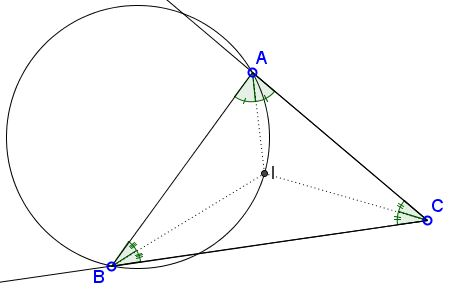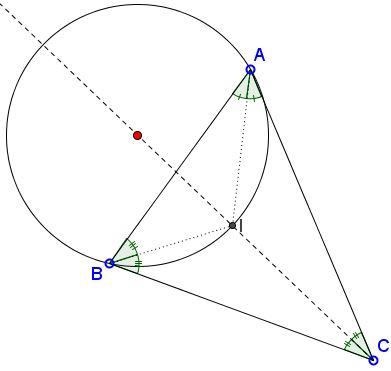# Properties of Circle Through the Incenter

Circle through two vertices and the incenter of a triangle has many attractive properties.Below I list a few (that I know of) with proofs or links to the pages that establish these properties. Every one's welcome to suggest either properties not listed here or alternative proofs to the one that are.

The center of $(ABI)$ lies on the bisector of $\angle ACB.$Proof

Let $X$ be the second intersection of $BC$ with $(ABI),$ $Y$ the second intersection of $AC$ with $(ABI).$Then $AX=BY.$

Proof

Point $E$ on circle $(ABI)$ through $A,B,I$ has the property that $BE=AB.$Then $\angle ABE=\angle ACB.$

Proof

The center $O_c$ of $(ABI)$ lies on the circumcircle $(ABC).$Proof

Let $BE$ be a chord in $(ABI)$ such that $BE=AB.$ Let $O$ be the circumcenter of $\Delta ABC.$Then $BE\perp BO.$

Proof